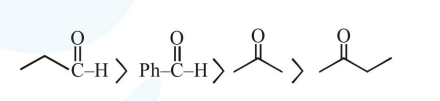# The increasing order of the reactivity of the following compound in nucleophilic addition reaction is :

Question:

The increasing order of the reactivity of the following compound in nucleophilic addition reaction is :

Propanal, Benzaldehyde, Propanone, Butanone

1. Butanone $<$ Propanone $<$ Benzaldehyde $<$

Propanal

2. Benzaldehyde $<$ Butanone $<$ Propanone $<$ Propanal

3. Propanal < Propanone < Butanone <

Benzaldehyde

4. Benzaldehyde $<$ Propanal $<$ Propanone $<$

Butanone

Correct Option: 1

Solution:

Reactivity order of various carbonyl compounds $\rightarrow$ Aldehydes $>$ Ketones